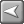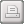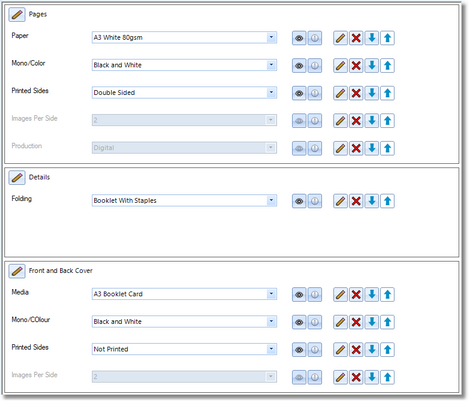﻿ A4 Booklet Example
Skyline Manual

# A4 Booklet Example# A4 Booklet ExampleThe example is for an A4 booklet. The printing is onto A3 paper which is folded and stapled to produce the booklet. The customer has the option of a different cover, but obviously the front and back cover will be the same media.

Points to remember:

 • The document option Images Per Side must always be included in the body/pages document part though It can be hidden from the end user.
 • The Body document part should always contain the document options Media, Printed Sides, Printer Type and Production.
 • The option Media should always be at the top of the list.
 • The option Production is usually hidden as the user is not required to select the type of printer to be used.If you are using CostManager with the costs entered as shown below, the cost for a 40 page booklet printed double sided with a printed single sided cover would be 3:50 per copy. It has also been assumed that there are no costs per document applied for creating the booklet. If an additional handling charge is required the binding cost per document for a booklet with staples could be added.The front and back cover cost is 2.06

A3 Booklet Card, printed single sided in colour.

Media cost is calculated by:
1 sheet of A3 booklet card is required at a cost of 2.00 per sheet
1 x 2.00 = 2.00

Printing cost is calculated by:
2 divided by 2 as images per side is 2 = 1
Printed single sided
1 x 0.06 = 0.06

The pages cost is 1.44

There are 38 pages printed double sided in black & white on A3 white paper 80gsm

Media cost is calculated by:
38 divided by 2 as images per side is 2 = 19.
19 divided by 2 being printed double sided = 9.5
Therefore 10 pages of A3 white paper 80gsm required at a cost of 0.03 per sheet.
10 x 0.03 = 0.30

Printing cost is calculated by:
38 divided by 2 as images per side is 2 = 19.
Printed double sided
Therefore 19 sides are being printed
19 x 0.06 = 1.14